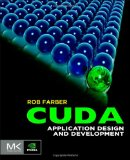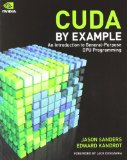# Matrix multiplication on GPU using CUDA with CUBLAS, CURAND and Thrust

## Posted on May 31, 2012 by Paul

The code for this tutorial is on GitHub: https://github.com/sol-prog/cuda_cublas_curand_thrust.

Matrix multiplication is an essential building block for numerous numerical algorithms, for this reason most numerical libraries implements matrix multiplication. One of the oldest and most used matrix multiplication implementation GEMM is found in the BLAS library. While the reference BLAS implementation is not particularly fast there are a number of third party optimized BLAS implementations like MKL from Intel, ACML from AMD or CUBLAS from NVIDIA.

In this post I’m going to show you how you can multiply two arrays on a CUDA device with CUBLAS. A typical approach to this will be to create three arrays on CPU (the host in CUDA terminology), initialize them, copy the arrays on GPU (the device on CUDA terminology), do the actual matrix multiplication on GPU and finally copy the result on CPU. Our first example will follow the above suggested algorithm, in a second example we are going to significantly simplify the low level memory manipulation required by CUDA using Thrust which aims to be a replacement for the C++ STL on GPU.

Let’s start by allocating space for our three arrays on CPU and GPU:

Please note the way in which we allocate memory for data on CPU using C’s malloc (line 10) and GPU using CUDA’s cudaMalloc (line 16), at the end of the main function we can free the device memory with cudaFree. The above code uses d_ for prefixing the arrays located on GPU and h_ for the host (CPU) arrays. We use 3x3 arrays in this example for simplicity, in a real application you should use much larger arrays for using the device efficiently.

Next step is to initialize the arrays A and B with some values, we are going to fill them with random numbers on the GPU using the CURAND library. In order to do this we could write a function that receives as input a device array, after the function is executed the array will be filled with random nubers:

If the arrays A and B are already filled with meaningful data, on CPU, or you simply want to fill them with random data on CPU and avoid the use of CURAND, you will need to transfer the host arrays to GPU:

As a side note, BLAS uses internally column-major order storage (Fortran order) and not the typical row-major storage used in C or C++ for multidimensional arrays.

Now that the arrays A and B are initialized and transfered on GPU we could write a function that will do the actual multiplication:

Observation - If you need to do more than one matrix multiplication in your code it is advisable to move the create/destroy handle code (lines 15 - 16 and 22) from the above function in the main function, and use the same handle for all multiplications. In this case the gpu_blas_mmul function became:

We can also implement an utility function for printing the result of the multiplication on the standard output:

Using the above pieces we present here for reference the complete main function:

For compiling the above example open a Terminal (Mac and Linux) or a Visual Studio Command Prompt window (for Windows) and write:

This is the result of running the code on my Mac:

As mentioned in the introduction, we could use Thrust to further simplify the above code. For example we could avoid completely the need to manually manage memory on the host and device using a Thrust vector for storing our data. Reimplementing the above example with Thrust will halve the number of lines of code from the main function:

Please note, see lines 11 12 21, the way in which we convert a Thrust device_vector to a CUDA device pointer.

If you are interested in learning CUDA, I would recommend reading CUDA Application Design and Development by Rob Farber.or CUDA by Example: An Introduction to General-Purpose GPU Programming by J. Sanders and E. Kandrot.